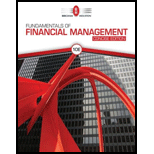# CURRENT YIELD, CAPITAL GAINS YIELD, AND YIELD TO MATURITY Pelzer Printing Inc. has bonds outstanding with 9 years left to maturity. The bonds have a 9% annual coupon rate and were issued 1 year ago at their par value of $1,000. However, due to changes in interest rates, the bond’s market price has fallen to$910.30. The capital gains yield last year was −8.97%. a. What is the yield to maturity? b. For the coming year, what are the expected current and capital gains yields? (Hint: Refer to footnote 7 for the definition of the current yield and to Table 7.1.) c. Will the actual realized yields be equal to the expected yields if interest rates change? If not, how will they differ?### Fundamentals Of Financial Manageme...

10th Edition
Eugene F. Brigham + 1 other
Publisher: Cengage Learning
ISBN: 9781337902571

#### Solutions

Chapter
Section### Fundamentals Of Financial Manageme...

10th Edition
Eugene F. Brigham + 1 other
Publisher: Cengage Learning
ISBN: 9781337902571
Chapter 7, Problem 10P
Textbook Problem
1 views

## CURRENT YIELD, CAPITAL GAINS YIELD, AND YIELD TO MATURITY Pelzer Printing Inc. has bonds outstanding with 9 years left to maturity. The bonds have a 9% annual coupon rate and were issued 1 year ago at their par value of $1,000. However, due to changes in interest rates, the bond’s market price has fallen to$910.30. The capital gains yield last year was −8.97%. a. What is the yield to maturity? b. For the coming year, what are the expected current and capital gains yields? (Hint: Refer to footnote 7 for the definition of the current yield and to Table 7.1.) c. Will the actual realized yields be equal to the expected yields if interest rates change? If not, how will they differ?

a.

Summary Introduction

To determine: Yield to maturity (YTM).

Yield to Maturity (YTM):

It refers to the rate of interest earned till the maturity of the bond by the bond holder.

### Explanation of Solution

Given,

Coupon rate is 9% or 0.09.

Selling price (value of bond) is $910.30. Par value of bond is$1,000.

Maturity is after 9 years.

Yield to maturity (YTM) can be computed through the bond value.

Formula to calculate present value of bond,

Bond'svalue=t=1NINT(1+rd

b.

Summary Introduction

To determine: Current yield and capital gain yields.

Current Yield: Current yield is the rate of return earned by the bond holders currently.

c.

Summary Introduction

To identify: Whether the actual realized yield be equivalent to the expected yields if there is a change in interest rates.

### Still sussing out bartleby?

Check out a sample textbook solution.

See a sample solution

#### The Solution to Your Study Problems

Bartleby provides explanations to thousands of textbook problems written by our experts, many with advanced degrees!

Get Started